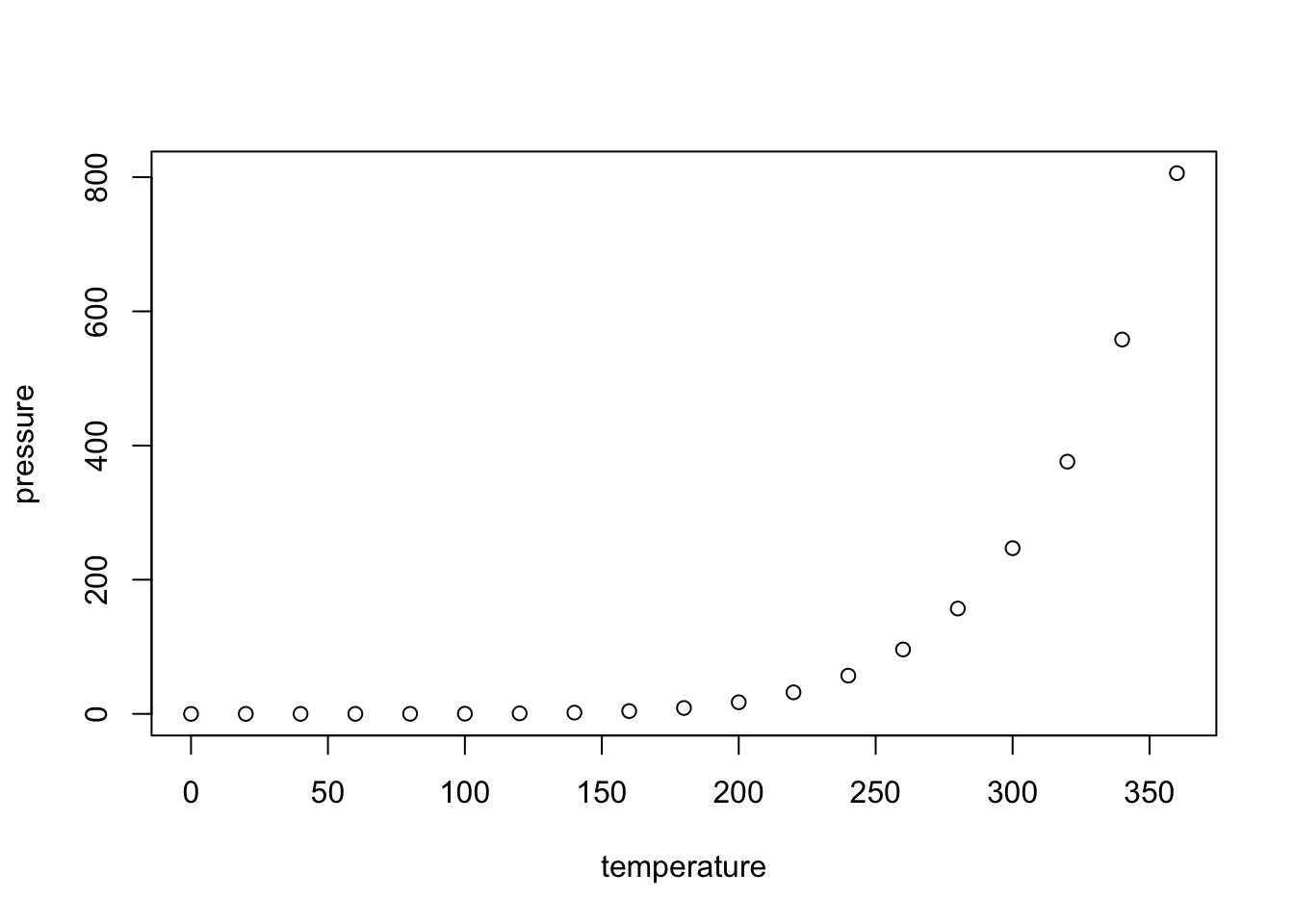R Markdown

This is an R Markdown document. Markdown is a simple formatting syntax for authoring HTML, PDF, and MS Word documents. For more details on using R Markdown see http://rmarkdown.rstudio.com.

When you click the Knit button a document will be generated that includes both content as well as the output of any embedded R code chunks within the document. You can embed an R code chunk like this:

summary(cars)
##      speed           dist
##  Min.   : 4.0   Min.   :  2.00
##  1st Qu.:12.0   1st Qu.: 26.00
##  Median :15.0   Median : 36.00
##  Mean   :15.4   Mean   : 42.98
##  3rd Qu.:19.0   3rd Qu.: 56.00
##  Max.   :25.0   Max.   :120.00

Including Plots

You can also embed plots, for example:Note that the echo = FALSE parameter was added to the code chunk to prevent printing of the R code that generated the plot.

Problem 1:

1. A discrete random variable $$X$$ has a probability mass function of the form $$P(X=x) =\frac{k}{2^x}$$ for $$x= 1,2,3$$ and zero otherwise, find $$k$$.

We know that the $$\sum_{x=1}^{3} \frac{k}{2^x} = 1$$. Therefore, we have that:

\begin{aligned} \sum_{x=1}^{3} \frac{k}{2^x} &= \frac{k}{2^1} + \frac{k}{2^2} + \frac{k}{2^3} \\ &= \frac{k}{2} + \frac{k}{4} + \frac{k}{8} \\ &= \frac{7k}{8}\\ \Rightarrow k = \frac{8}{7} \\ \square \end{aligned}

1. Can a function of the form $$f(x)=c(2^{-x}-0.5)$$ for $$x= 0,1,2$$ and zero otherwise be a probability mass function of a random variable?

$$f(0)=c(2^{-0}-0.5)= .50c \\$$ $$f(1)=c(2^{-1}-0.5)= 0 \\$$ $$f(2)=c(2^{-2}-0.5)=-.25c \\$$ $$f(0) + f(1) + f(2) = .25 \\$$ $$\Rightarrow c = 4 \\$$

However, we know that $$f(2)=4(2^{-2}-0.5)=-1$$ Which is a contradiction since for a pdf $$f(x) \geq 0$$. Thus $$f(x)$$ is not a pdf $$\square$$

Problem 2: A function of the form $$f(t)=ct^{-c-1}I\{t >1\}$$ for $$t \in (-\infty,\infty)$$.

1. If $$f(t)$$ is a probability density function, find the value of $$c$$.

We use the definition of a pdf to show that:

\begin{aligned} \int_{-\infty}^{\infty} f(t) dt &= 1 \\ \int_{-\infty}^{\infty} f(t) dt &= \int_{-\infty}^{\infty} ct^{-c-1}I\{t >1\} dt \\ &= \int_{1}^{\infty} ct^{-c-1} dt \\ &= -t^{-c} \Big|_1^\infty \\ &= \frac{1}{\infty^c} + 1^{-c} \\ \Rightarrow c > 0 \end{aligned} We know this since if $$c = 0$$ then we would get that $$f(t)=0 \Rightarrow \int_{-\infty}^{\infty} f(t)tx = \int_{-\infty}^{\infty} 0dt=0$$ which is a contradiction of the definition of a pdf.

Furthermore if we have $$c < 0$$ then we would get that $$\frac{1}{\infty^c} + 1^{-c} = \infty$$, which also contradicts the definition of a pdf and that $$\int_{-\infty}^{\infty} f(t)dt = 1 \square$$.

1. Find the corresponding cumulative distribution function of $$f(t)$$ in (1).

\begin{aligned} P(T \leq t) &= F_T(t) \\ &= \int_{-\infty}^{t} f_T(x) dx \\ &= \int_{-\infty}^{t} cx^{-c-1}I\{x >1\} dx \\ &= \int_{1}^{t} cx^{-c-1} dx \\ &= -x^{-c} \Big|_1^t \\ &= -t^{-c} + 1^{-c} \\ &= (1-\frac{1}{t^c})I\{t >1\} \end{aligned}

Problem 3: Suppose $$f(t)$$ and $$g(t)$$ for $$t \in (-\infty,\infty)$$ are probability density functions. Let $$a \geq 0$$ and $$b \geq 0$$ are two fixed constants satisfying $$a + b = 1$$. Prove that $$af(t) + bg(t)$$ is also a probability density function for $$t \in (-\infty,\infty)$$.

To show that $$af(t) + bg(t)$$ is a pdf we prove that $$af(t) + bg(t) \geq 0, \forall x$$ and $$\int_{-\infty}^{\infty} af(t) + bg(t) dt = 1$$.

1. We first show that $$af(t) + bg(t) \geq 0$$:

Assume that there exist a t such that $$af(t) + bg(t) < 0$$. This implies that at least one of the two terms is the function are negative. From this we know that if $$af(t) < 0$$ either $$a < 0$$ or $$f(t) < 0$$ which is a contradiction since we know that $$f(t) \geq 0$$ (by the definition of a pdf) and $$a>0$$ by the statement of the problem. If $$bg(t) < 0$$ then either $$b < 0$$ or $$g(t) < 0$$ which is also a contradiction, since we know that $$g(t) \geq 0$$ (by the definition of a pdf) and $$b>0$$ by the statement of the problem. Therefore by contradiction, we know that $$af(t) + bg(t) \geq 0$$

1. We show that $$\int_{-\infty}^{\infty} (af(t) + bg(t)) dx = 1$$

\begin{aligned} \int_{-\infty}^{\infty} (af(t) + bg(t)) dt &= \int_{-\infty}^{\infty} af(t)dt + \int_{-\infty}^{\infty}bg(t) dx \\ &= a\int_{-\infty}^{\infty} f(t)dt + b\int_{-\infty}^{\infty}g(t) dx \\ &= a(1) + b(1), \space \space \space \space \space \space \space \space \text{since the Defintion of pdf we know that} \int_{-\infty}^{\infty} f(t)dt = 1, \int_{-\infty}^{\infty} g(t)dt = 1\\ &= a + b \\ &= 1 \\ \end{aligned}

From this we know that the definition of a pdf holds for $$af(t)+bg(t)$$. $$\square$$